# Pseudo-prime

(diff) ← Older revision | Latest revision (diff) | Newer revision → (diff)

Traditionally, a composite natural numberis called a pseudo-prime ifmodulo, for it has long been known that primes have this property. (The term is apparently due to D.H. Lehmer.) There are infinitely many such, the first five being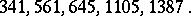More recently, the concept has been extended to include any composite number that acts like a prime in some realization of a probabilistic primality test. That is, it satisfies some easily computable necessary, but not sufficient, condition for primality. Pseudo-primes in this larger sense include:

1) ordinary base-pseudo-primes, satisfying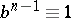modulo;

2) Euler base-pseudo-primes, whose Jacobi symbol withsatisfies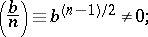3) strong base-pseudo-primes, for which the sequencemodulo,, is either always, or contains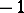. (Herewithodd.)

For each, the implications 3)2)1) hold. A numberthat is an ordinary base-pseudo-prime for allprime tois called a Carmichael number. Analogous numbers for the other two categories do not exist.

For a thorough empirical study of pseudo-primes, see [a4]. Lists of pseudo-primes to various small bases can be found in [a6].

The concept of a pseudo-prime has been generalized to include primality tests based on finite fields and elliptic curves (cf. also Finite field; Elliptic curve). For reviews of this work, see [a3], [a5].

The complementary concept is also of interest. The baseis called a (Fermat) witness forifis composite but not a base-pseudo-prime. Euler and strong witnesses are similarly defined. If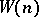, the smallest strong witness for, grows sufficiently slowly, there is a polynomial-time algorithm for primality. It is known thatis not bounded [a2], but if an extended version of the Riemann hypothesis (cf. Riemann hypotheses) holds, then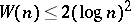[a1].

How to Cite This Entry:
Pseudo-prime. Encyclopedia of Mathematics. URL: http://encyclopediaofmath.org/index.php?title=Pseudo-prime&oldid=16100
This article was adapted from an original article by E. Bach (originator), which appeared in Encyclopedia of Mathematics - ISBN 1402006098. See original article